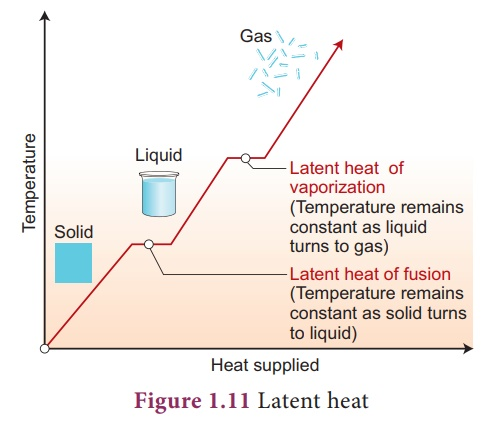Home | | Science 9th std | Latent Heat

# Latent Heat

The word, ‘latent’ means hidden. So, latent heat means hidden heat or hidden energy. In order to understand latent heat, let us do the activity given below.

Latent Heat

The word, ‘latent’ means hidden. So, latent heat means hidden heat or hidden energy. In order to understand latent heat, let us do the activity given below.

In the above activity, the temperature is constant at 0˚C until entire ice is converted into liquid and again constant at 100˚C until all the ice is converted into vapor. Why? It is because, when a substance changes from one state to another, a considerable amount of heat energy is absorbed or liberated. is energy is called latent heat. us, latent heat is the amount of heat energy absorbed or released by a substance during a change in its physical sates without any change in its temperature.

Heat energy is absorbed by a solid during melting and an equal amount of heat energy is liberated by the liquid during freezing, without any temperature change. It is called latent heat of fusion. In the same manner, heat energy is absorbed by a liquid during vaporization and an equal amount of heat energy is liberated by the vapor during condensation, without any temperature changes. is is called latent heat of vaporization.## Specific latent heat

Latent heat when expressed per unit mass of a substance, it is called specific latent heat. It is denoted by the symbol L. If Q is the amount of heat energy absorbed or liberated by m mass of a substance during its change of phase at a constant temperature, then specific latent heat is given as L = Q/m.

Thus, specific latent heat is the amount of heat energy absorbed or liberated by unit mass of a substance during change of state without causing any change in temperature. The SI unit of specific latent heat is J/kg.

### Exercise 1.6

How much heat energy is required to melt 5 kg of ice? (Speci c latent heat of ice = 336 Jg-1)

### Solution:

Given, m = 5 Kg = 5000g, L 336 Jg-1

Heat energy required m x L

= 5000 x 336

= 1680000J or 1.68 x 106 J

### Exercise 1.6

How much boiling water at 100ºC is needed to melt 2kg of ice so that the mixture which is all water is at 0ºC?

[Speci c heat capacity of water 4.2 JKg-1 and speci c latent heat of ice 336 Jg-1].

### Solution:

Given, Mass of ice = 2 kg = 2000 g.

Let m be the mass of boiling water required.

Heat lost = Heat gained.

m x c x Δt = m x L

m x 4.2 x (100-0) = 2000 x 336

m = 2000x336 / 4.2x100

= 1600g or 1.6 kg.

Study Material, Lecturing Notes, Assignment, Reference, Wiki description explanation, brief detail
9th Science : Heat : Latent Heat |